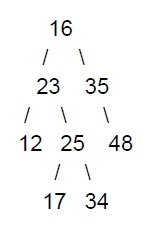# Program to print root to leaf paths without using recursion using C++

In this tutorial, we will be discussing a program to print the path from the root node to all the leaf nodes in a given binary tree.

For example, let us say we have the following binary treeIn this binary tree, we have 4 leaf nodes. Therefore we can have 4 paths from the root node to the leaf node.

To solve this, we will use the iterative approach. While doing preorder traversal of the binary tree we can store parent pointers in a map. Whenever during traversal we encounter a leaf node we can easily print its path from the root node using parent pointers.

## Example

#include <bits/stdc++.h>>
using namespace std;
struct Node{
int data;
struct Node *left, *right;
};
//to create a new node
Node* create_node(int data){
Node* node = new Node;
node->data = data;
node->left = node->right = NULL;
return node;
}
//printing the path from root to leaf
void print_cpath(Node* curr, map<Node*, Node*> parent){
stack<Node*> nodes_stack;
while (curr){
nodes_stack.push(curr);
curr = parent[curr];
}
while (!nodes_stack.empty()){
curr = nodes_stack.top();
nodes_stack.pop();
cout << curr->data << " ";
}
cout << endl;
}
//to perform pre order traversal
void preorder_traversal(Node* root){
if (root == NULL)
return;
stack<Node*> nodeStack;
nodeStack.push(root);
map<Node*, Node*> parent;
parent[root] = NULL;
while (!nodeStack.empty()){
Node* current = nodeStack.top();
nodeStack.pop();
if (!(current->left) && !(current->right))
print_cpath(current, parent);
if (current->right){
parent[current->right] = current;
nodeStack.push(current->right);
}
if (current->left){
parent[current->left] = current;
nodeStack.push(current->left);
}
}
}
int main(){
Node* root = create_node(101);
root->left = create_node(82);
root->right = create_node(23);
root->left->left = create_node(34);
root->left->right = create_node(55);
root->right->left = create_node(29);
preorder_traversal(root);
return 0;
}

## Output

101 82 34
101 82 55
101 23 29